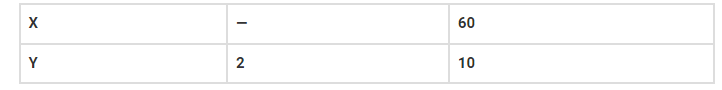# If x varies inversely as y,

Question:

If x varies inversely as y, thenSolution:

If x varies inversely as y, then;

x×y = k

If x = 60 and y = 10

Then, x×y = 60×10 = 600 = k

Hence, x×2 = 600

x = 600/2 = 300